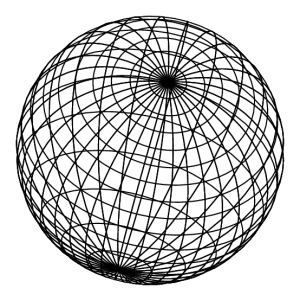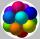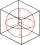# Cube in ball

Cube is inscribed into the sphere of radius 181 dm.

How many percent is the volume of
cube of the volume of the sphere?

p =  36.7553 %

### Step-by-step explanation:Did you find an error or inaccuracy? Feel free to write us. Thank you!Tips to related online calculators
Tip: Our volume units converter will help you with the conversion of volume units.

#### You need to know the following knowledge to solve this word math problem:

We encourage you to watch this tutorial video on this math problem:

## Related math problems and questions:

• Cube in sphereThe cube is inscribed in a sphere with a radius r = 6 cm. What percentage is the volume of the cube from the volume of the ball?
• Inscribed sphereHow many percent of the cube volume takes the sphere inscribed into it?
• Sphere vs cubeHow many % of the surface of a sphere of radius 12 cm is the surface of a cube inscribed in this sphere?
• Inscribed sphereHow many % of the volume of the cube whose edge is 6 meters long is a volume of a sphere inscribed in that cube?
• The ballThe ball has a radius of 2m. What percentage of the surface and volume is another sphere whose radius is 20% larger?
• Cube into sphereThe cube has brushed a sphere as large as possible. Determine how much percent the waste was.
• Body diagonalCalculate the cube volume, whose body diagonal size is 75 dm. Draw a picture and highlight the body diagonal.
• Cube in sphereThe sphere is an inscribed cube with an edge of 8 cm. Find the sphere's radius.
• Cube and sphereCube with the surface area 150 cm2 is described sphere. What is sphere surface?
• EightEight small Christmas balls with a radius of 1 cm have the same volume as one large Christmas ball. What has a bigger surface: eight small balls, or one big ball?
• Reverse Pythagorean theoremGiven are lengths of the sides of the triangles. Decide which one is rectangular: Δ ABC: 77 dm, 85 dm, 36 dm ... Δ DEF: 55 dm, 82 dm, 61 dm ... Δ GHI: 24 mm, 25 mm, 7 mm ... Δ JKL: 32 dm, 51 dm, 82 dm ... Δ MNO: 51 dm, 45 dm,
• Sphere fallHow many percent fall volume of a sphere if diameter decreases 3×?
• Spheres in sphereHow many spheres with a radius of 15 cm can fit into the larger sphere with a radius of 150 cm?The radius of the sphere we reduce by 1/3 of the original radius. How much percent does the volume and surface of the sphere change?What was the cube's original edge length after cutting 39 small cubes with an edge of 2 dm left 200 dm3?The cube is inscribed in a sphere with a volume 7253 cm3. Determine the length of the edges of a cube.A circle is inscribed at the bottom wall of the cube with an edge (a = 1). What is the radius of the spherical surface that contains this circle and one of the vertex of the top cube base?